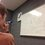Constructing Mathematics

There have been several threads lately about "constructing" our mathematical numbers and operations. I wanted to create a new discussion in hopes of getting the brilliant community actively engaged in a foundational talk about mathematics. I take most of the following questions from Calvin's comment in this thread. You may want to read up on this thread and the recent thinking like a theorist. Here are the things to think about:

1) When we are first introduced to addition and multiplication, we can only add finitely many of them together. What does an infinite summation (or multiplication) represent? Is it 'legal'? Does it obey the commutative and distributive laws?

2) What is the concept of infinity? Is it simply "bigger than any number"? Are there different concepts of infinity? How does infinity affect addition and multiplication? What is ∞/∞? Which is 'bigger', ∞+∞ or ∞×∞?

3) What is addition and multiplication? Are there other ways that we can 'add' and 'multiply' in other systems (say colors)? What is the essential characteristics / qualities of addition and multiplication?

4) Is there mathematics that no system can handle? Can we prove or disprove every statement?

5) Is there an 'innate' idea of mathematics? Certain concepts which we take as obvious truths actually took a long time to develop. The concept of 0 was only introduced in 9th century AD by India. Using symbols to represent equations (i.e. algebra in high school) only developed in 16-17th century.

6) Is there math that an isolated civilization will never discover because of the way that they decide to interpret the world? How different is the world if we started off learning about complex numbers immediately?

7) Is there more than one way to approach mathematics? With set theory, logic, intuitively, algebraicly, geometrically, practically, etc. Is there a best way?

I hope these questions spark a few thoughts in all of your brains. If you have any other interesting questions, feel free to comment away. Post your thoughts below.Note by Bob Krueger
6 years, 3 months ago

This discussion board is a place to discuss our Daily Challenges and the math and science related to those challenges. Explanations are more than just a solution — they should explain the steps and thinking strategies that you used to obtain the solution. Comments should further the discussion of math and science.

When posting on Brilliant:

• Use the emojis to react to an explanation, whether you're congratulating a job well done , or just really confused .
• Ask specific questions about the challenge or the steps in somebody's explanation. Well-posed questions can add a lot to the discussion, but posting "I don't understand!" doesn't help anyone.
• Try to contribute something new to the discussion, whether it is an extension, generalization or other idea related to the challenge.
• Stay on topic — we're all here to learn more about math and science, not to hear about your favorite get-rich-quick scheme or current world events.

MarkdownAppears as
*italics* or _italics_ italics
**bold** or __bold__ bold

- bulleted
- list

• bulleted
• list

1. numbered
2. list

1. numbered
2. list
Note: you must add a full line of space before and after lists for them to show up correctly
paragraph 1

paragraph 2

paragraph 1

paragraph 2

> This is a quote
This is a quote
# I indented these lines
# 4 spaces, and now they show
# up as a code block.

print "hello world"
# I indented these lines
# 4 spaces, and now they show
# up as a code block.

print "hello world"
MathAppears as
Remember to wrap math in $$...$$ or $...$ to ensure proper formatting.
2 \times 3 $2 \times 3$
2^{34} $2^{34}$
a_{i-1} $a_{i-1}$
\frac{2}{3} $\frac{2}{3}$
\sqrt{2} $\sqrt{2}$
\sum_{i=1}^3 $\sum_{i=1}^3$
\sin \theta $\sin \theta$
\boxed{123} $\boxed{123}$

Sort by:

This is just to let you know that we are planning to adapt some of these questions for the future discussions in the "Understanding Mathematics" series. Stay tuned!

Staff - 6 years, 3 months ago